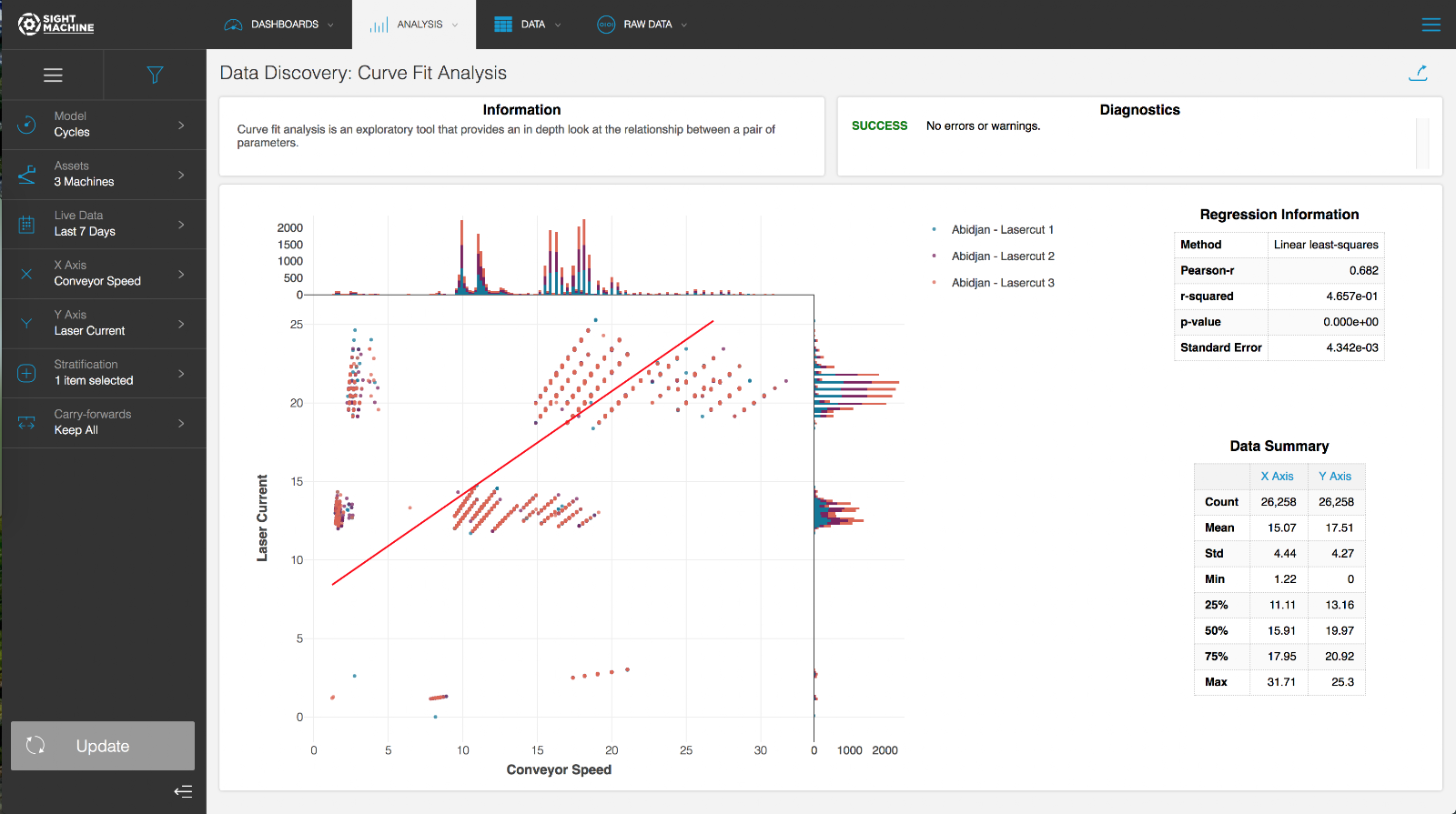Curve Fit AnalysisUse Cases

The Curve Fit Analysis is designed for investigating the details of the relationship between two parameters. Even though two parameters may have a high correlation value, this may be an artifact of the data shape rather than an indication of a meaningful relationship that can be put to use. The Curve Fit Analysis is most helpful later in the exploratory process, after you have identified a pair of parameters to analyze in detail. This is a good tool to follow the Correlation Heatmap or Time-Series Correlation.

Specifications

The Curve Fit Analysis is a multivariate analysis that you can perform on continuous fields on the Cycle and Part models. You can select multiple machines of a given type when performing the analysis on the Cycle model. Using the Cycle model, the Curve Fit Analysis can investigate parameters from the same machine type with high granularity. Using the Part model lets you compare fields across different machines, but with lower granularity (the Part model is limited by the serial number or other identifier used to construct the data model).

Analysis

The Curve Fit Analysis is an exploratory tool that allows you to investigate the relationship between a pair of parameters by computing a linear least-squares regression for the parameters. The analysis plots the selected points on a scatter plot, with a histogram along each axis to show the parameters’ distributions, as well as the fit line determined by the regression. You can choose a categorical field for stratification, which breaks the points into separate traces for each value in the category. The Curve Fit Analysis provides a table of metrics for the regression, including the Pearson-R correlation coefficient and r-squared. This tool also presents a table of summary statistics for each selected parameter that includes Count, Standard Deviation, Minimum, and Maximum.